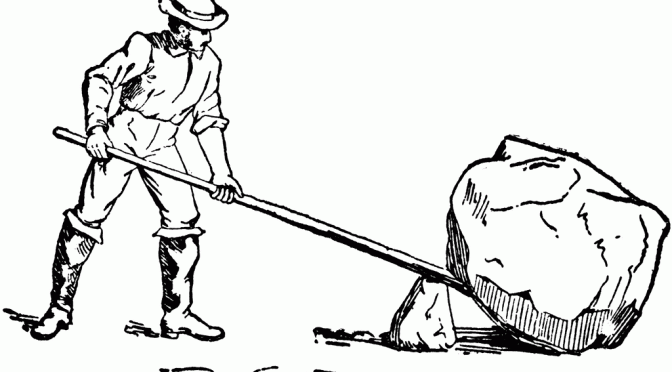# Chronologia mechaniki klasycznej

 -260 Archimedes mathematically works out the principle of the lever and discovers the principle of buoyancy. 60 Hero of Alexandria writes Metrica, Mechanics, and Pneumatics, 1490 Leonardo da Vinci describes capillary action. 1581 Galileo Galilei notices the timekeeping property of the pendulum. 1589 Galileo Galilei uses balls rolling on inclined planes to show that different weights fall with the same acceleration. 1638 Galileo Galilei publishes Dialogues Concerning Two New Sciences. 1658 Christian Huygens experimentally discovers that balls placed anywhere inside an inverted cycloid reach the lowest point of the cycloid in the same time and thereby experimentally shows that the cycloid is the isochrone. 1668 John Wallis suggests the law of conservation of momentum. 1687 Isaac Newton publishes his Principia Mathematica. 1690 James Bernoulli shows that the cycloid is the solution to the isochrone problem. 1691 Johann Bernoulli shows that a chain freely suspended from two points will form a catenary. 1691 James Bernoulli shows that the catenary curve has the lowest center of gravity that any chain hung from two fixed points can have. 1696 Johann Bernoulli shows that the cycloid is the solution to the brachistochrone problem. 1714 Brook Taylor derives the fundamental frequency of a stretched vibrating string in terms of its tension and mass per unit length by solving an ordinary differential equation. 1733 Daniel Bernoulli derives the fundamental frequency and harmonics of a hanging chain by solving an ordinary differential equation. 1734 Daniel Bernoulli solves the ordinary differental equation for the vibrations of an elastic bar clamped at one end. 1738 Daniel Bernoulli examines fluid flow in Hydrodynamica. 1739 Leonhard Euler solves the ordinary differential equation for a forced harmonic oscillator and notices the resonance phenomenon. 1742 Colin Maclaurin discovers his uniformly rotating self-gravitating spheroids. 1747 Pierre-Louis Moreau de Maupertuis applies minimum principles to mechanics. 1759 Leonhard Euler solves the partial differential equation for the vibration of a rectangular drum. 1764 Leonhard Euler examines the partial differential equation for the vibration of a circular drum and finds one of the Bessel function solutions. 1788 Joseph Lagrange presents Lagrange’s equations of motion in Mecanique Analytique. 1789 Antoine Lavoisier states the law of conservation of mass. 1821 William Hamilton begins his analysis of Hamilton’s characteristic function. 1834 Carl Jacobi discovers his uniformly rotating self-gravitating ellipsoids. 1834 John Russell observes a nondecaying solitary water wave in the Union Canal near Edinburgh and uses a water tank to study the dependence of solitary water wave velocities on wave amplitude and water depth. 1835 William Hamilton states Hamilton’s canonical equations of motion. 1835 Gaspard de Coriolis examines motion on a spinning surface deduces the Coriolis effect. 1842 Christian Doppler examines the Doppler shift of sound. 1847 Hermann Helmholtz formally states the law of conservation of energy. 1851 Jean-Bernard Foucault shows the Earth’s rotation with a huge pendulum. 1902 James Jeans finds the length scale required for gravitational pertrubatations to grow in a static nearly homogeneous medium.

Za The Chronology of Events in Science, Mathematics, and Technology